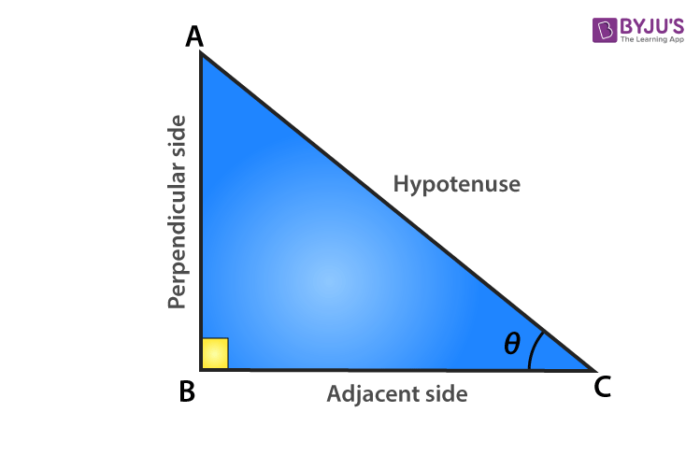What are the six trig identities

The six trigonometric identities or the trigonometric functions are Sine, Cosine, Tangent, Secant, Cosecant and Cotangent. They are abbreviated as sin, cos, tan, sec, cosec and cot. Among the six trig functions, sin, cos and tan are primary functions, whereas sec, cosec and cot are the derived functions from the primary one.

In trigonometry, we learn the relation between angles and sides of a right-triangle. The six trig identities are also called trigonometric ratios because they can be represented as the ratio of two sides of a right triangle, with respect to a specific angle (acute). Let us consider a right-angled triangle ABC, given below.As we can see, in ∆ABC, there are three sides, such as;

AB = Perpendicular/Opposite side to angle θ

BC = Base/Adjacent Side to angle θ

AC = Hypotenuse side/side opposite to right angle

Now, with the help of the above triangle, we can state the relationship between trigonometric ratios and sides of triangle:

• Sin θ = Opposite side/Hypotenuse
• Cos θ = Adjacent Side/Hypotenuse
• Tan θ = Opposite side/Adjacent Side
• Sec θ = 1/cos θ = Hypotenuse/Adjacent Side
• Cosec θ = 1/Sin θ = Hypotenuse/Opposite side
• Cot θ = 1/tan θ = Adjacent Side/Opposite side

Hence, we have known the six trigonometric functions and their formulas as well. Also, if we know the sides of the right triangle, then we can easily determine all the trigonometry ratios.

Example: A right triangle has sides, such that;

Opposite sides, AB = 3cm

Hypotenuse, AC = 5 cm

Find the value of all trigonometry identities.

Solution:

Let the respective angle is θ. Therefore,

Sin θ = ⅗

Cos θ = ⅘

Tan θ = ¾

Sec θ = 5/4

Cosec θ = 5/3

Cot θ = 4/3

Hence, all the trig ratios are evaluated.(0)(0)